# Even Function

Even Function

The teacher asked the students to observe the following shapes and find the kind of symmetry seen in them.

Luke said that the shapes looked to be vertically symmetrical.

So, let us study the two types of functions, on the basis of their symmetry, to learn more about the concept.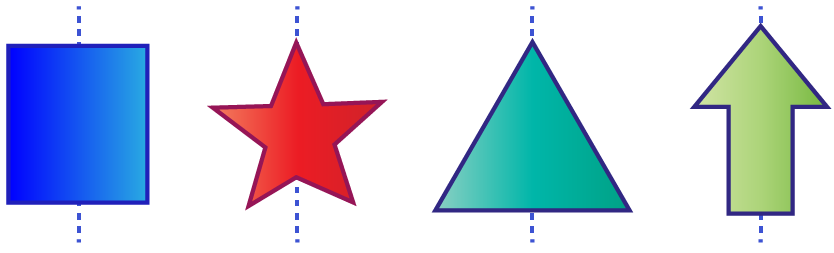## Lesson Plan

 1 Classification of Functions 2 What Is an Even Function? 3 Important Notes on Even Function 4 Solved Examples on Even Function 5 Interactive Questions on Even Function

## Classification of Functions

Functions can be even, odd, both, or neither of them.

Let us explore some even and odd function examples in this page.

### Even Function

A function is even if $$f(x) = f( - x)$$  for all $$x$$

This means that the function is the same for $$\text{+ve } x\text{-axis}$$ and $$\text{-ve } x\text{-axis,}$$ or graphically, symmetric about the $$y\text{-axis}$$.

For example, $$f(x) = {x^2}$$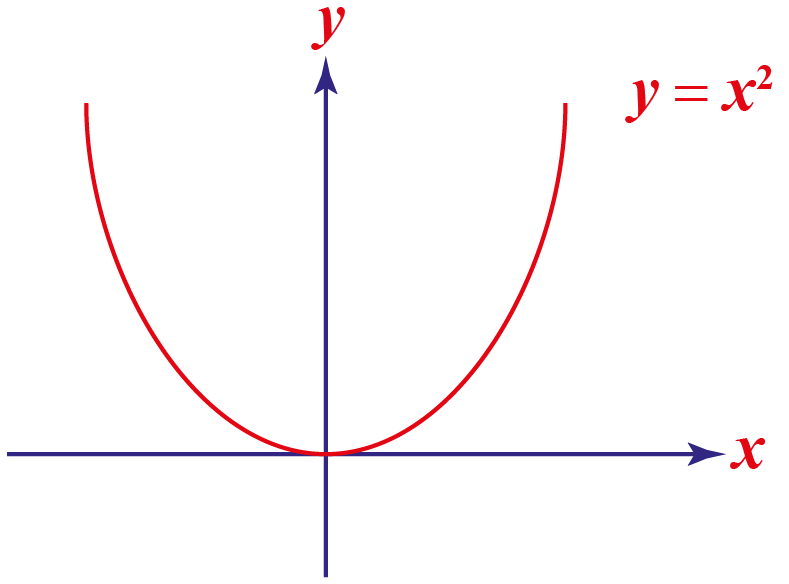### Odd Function

A function is odd if $$-f(x)= f(-x)$$  for all $$x$$.

The graph of an odd function is symmetrical about the origin.

For example, $$f(x) = {x^3}$$ is odd.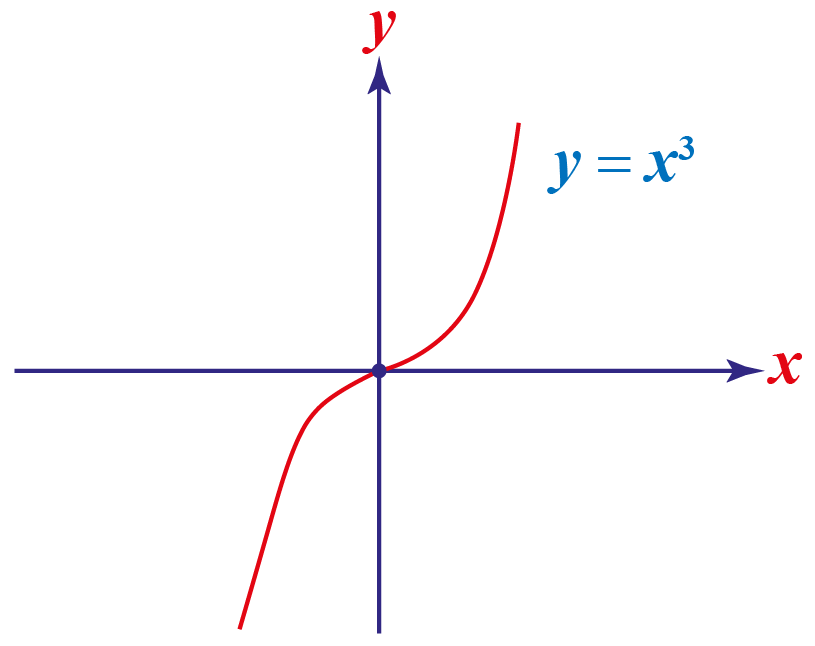### Neither Even, Nor Odd

A function $$f(x)$$  which is $$f(x) \neq f( - x)$$ and  $$-f(x) \neq f(-x)$$  for all $$x$$   is neither an even function, nor an odd function.

For example, $$f(x)=2x^{5}+3x^{2}+1$$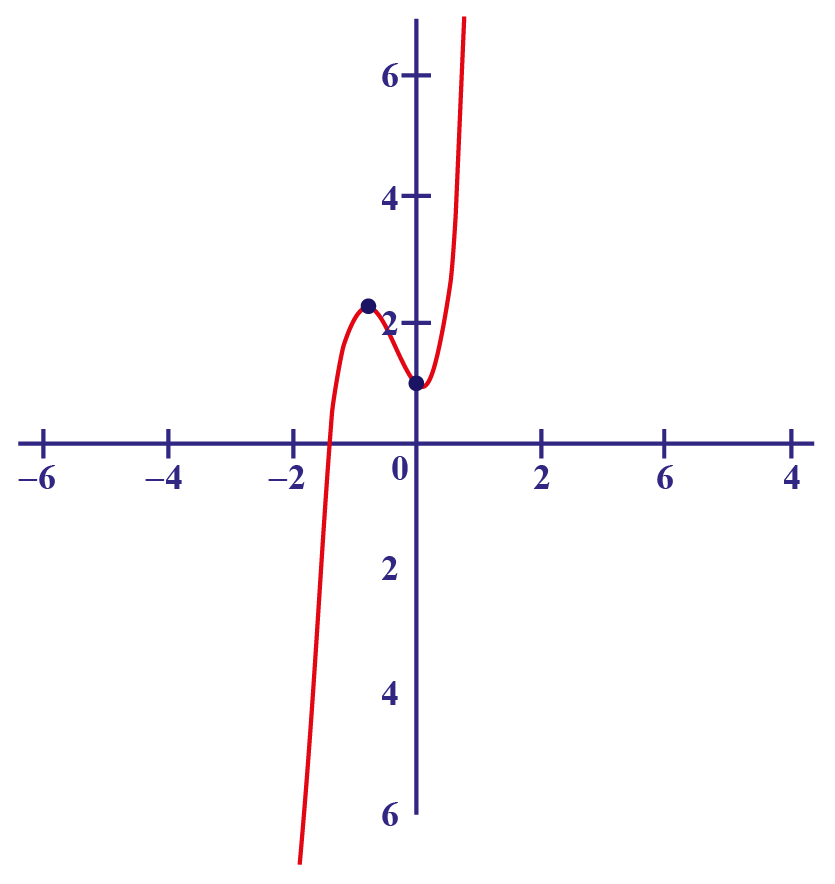We can see that the graph is not symmetrical about the origin or the y-axis.

### Both Odd and Even

A function is both odd and even, if  $$-f(x)= f(-x)$$  and  $$f(x) = f( - x)$$  for all $$x$$ .

The only function which is both odd and even is $$f(x) = 0$$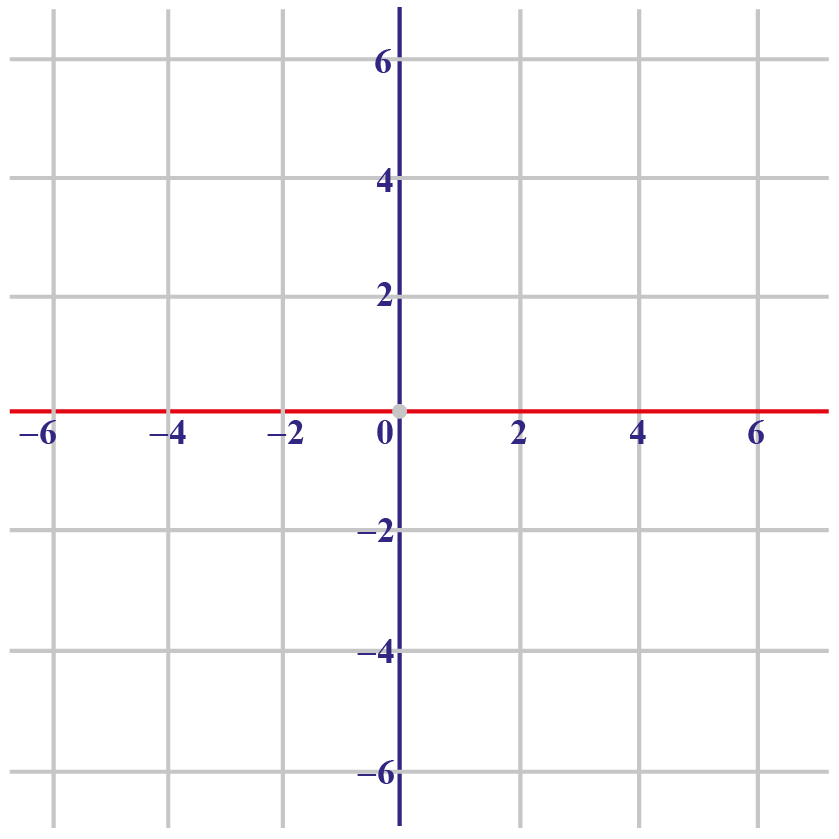The graph is symmetrical about the origin and y-axis.

## What Is an Even Function?

Let us first understand the meaning of even functions, algebraically.

A function is even if $$f(x) = f( - x)$$ for all values of $$x$$.

This means that the function is the same for $$\text{+ve } x\text{-axis}$$ and $$\text{-ve } x\text{-axis,}$$ or graphically, symmetric about the $$y\text{-axis}$$.

Now, let us see what this means.

For an even function $$f(x$$), if we plug in $$-x$$ in place of $$x$$, then the value of $$f(-x)$$ is equal to the value of $$f(x$$).

### Formula of Even Function

 $$f(-x)=f(x)$$ for all $$x$$

### Examples of Even Functions

1. Consider the function $$f(x)=x^2$$

Determine the value of $$f(-x)$$.

$$f(-x)=(-x)^2=x^2=f(x)$$

Therefore, $$f(x)=x^2$$ is an even function.

We can verify by taking a particular value of $$x$$.

For $$x=2$$, the value of $$f(x$$) is given by:

$$f(2)=2^2=4$$

The value of $$f(-x)$$ is given by:

$$f(-2)=(-2)^2=4=f(2)$$

Similarly, functions like $$x^4, x^6, x^8, x^{10}$$, etc. are even functions.

Interestingly, the above functions have even powers.

2. Consider a trigonometric function $$f(x)=\cos x$$

Determine the value of $$f(-x)$$.

$$f(-x)=\cos (-x)=\cos x=f(x)$$

$$\cos (-x)=\cos x$$ for all values of $$x$$

Therefore, $$f(x)=\cos x$$ is an even function.

Later, in this section, we will explore the properties of even functions and their graphical representation.Tips and Tricks
1. If the value of $$f(-x)$$ is same as the value of $$f(x)$$ for every value of $$x$$, the function is even.
2. If the value of $$f(-x)$$ is NOT the same as the value of $$f(x)$$ for any value of $$x$$, the function is not even.
3. If a function has an even power, the function need not be an even function.

## Graphical Representation of an Even Function

Let us now see how an even function behaves graphically.

The graph of an even function is symmetric with respect to the y-axis.

In other words, the graph of an even function remains the same after reflection about the y-axis.

Here are a few examples of even functions, observe the symmetry about the y-axis.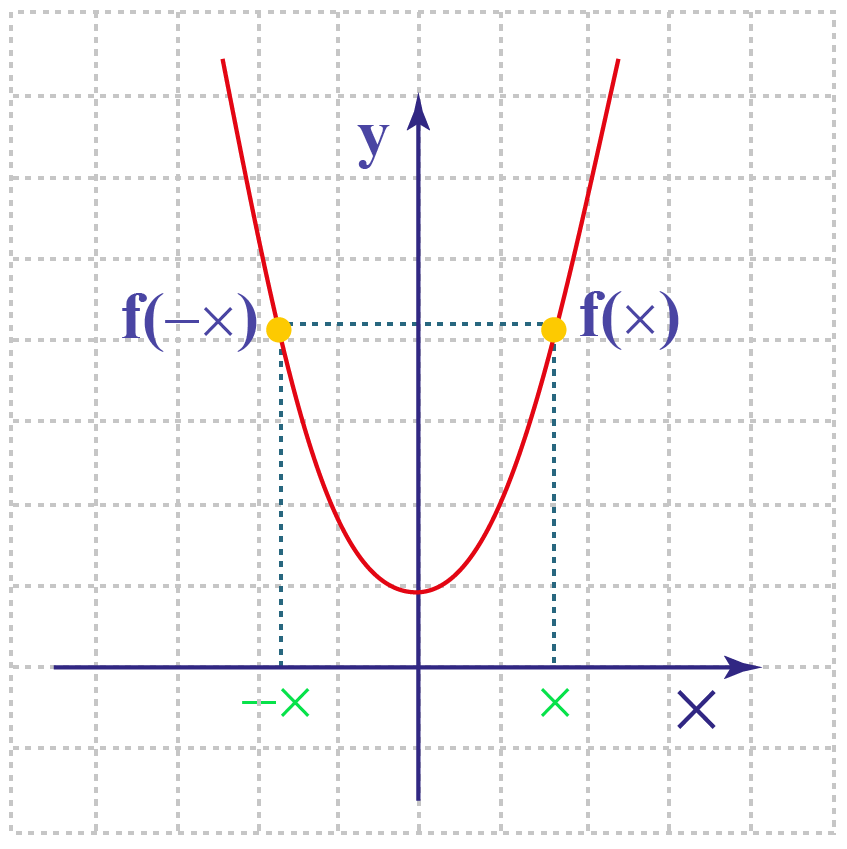### Example

$$y=x^2$$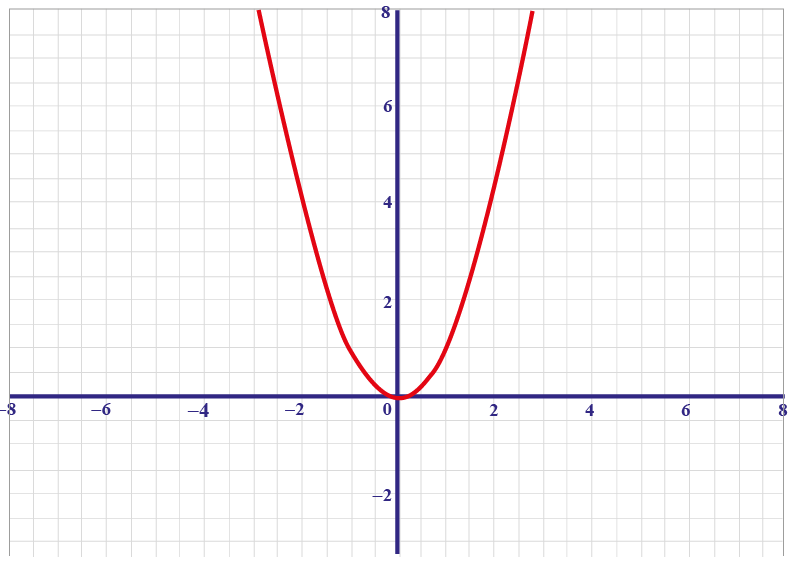$$f(x)=\cos x$$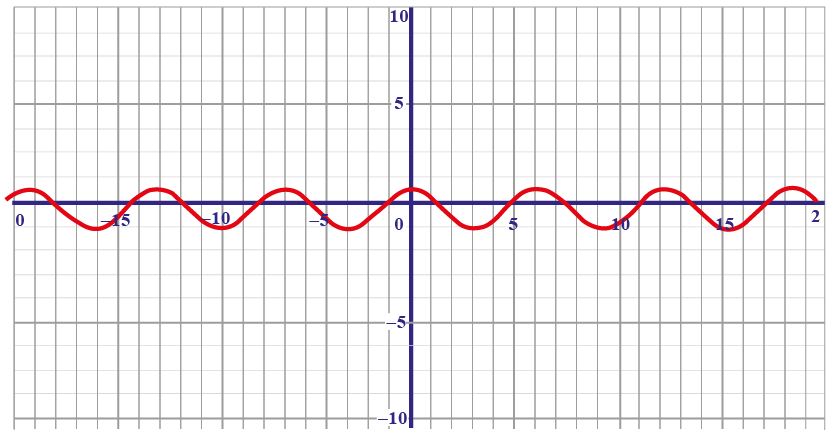More Important Topics
Numbers
Algebra
Geometry
Measurement
Money
Data
Trigonometry
Calculus
More Important Topics
Numbers
Algebra
Geometry
Measurement
Money
Data
Trigonometry
Calculus
Learn from the best math teachers and top your exams

• Live one on one classroom and doubt clearing
• Practice worksheets in and after class for conceptual clarity
• Personalized curriculum to keep up with school